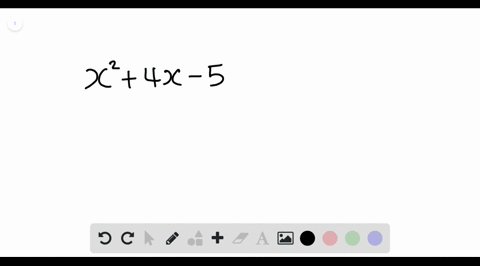Enroll in one of our FREE online STEM summer camps. Space is limited so join now!View Summer Courses### Factor each polynomial. If a polynomial cannot be…

00:49Other Schools

Need more help? Fill out this quick form to get professional live tutoring.

Get live tutoring
Problem 5

Factor each polynomial. If a polynomial cannot be factored, write prime. Factor out the greatest common factor as necessary.
$$m^{2}-5 m-14$$

$m^{2}-5 m-14=(m-7)(m+2)$

## Discussion

You must be signed in to discuss.

## Video Transcript

we're asked to factor this. Try no meal here. I know that the leading coefficient is one, so both factors must start with them Now. The constant term must multiply together to be negative 14 and add together to be negative five So we can list arm factors of negative. 14 impairs It'll be negative. One 14 Negative to positive. Seven Negative seven Positive too and negative. 14 Positive one. So out of these factors that we just listed Onley, this one here will add together to be negative five. So this means air factors would be on minus seven and em plus two.